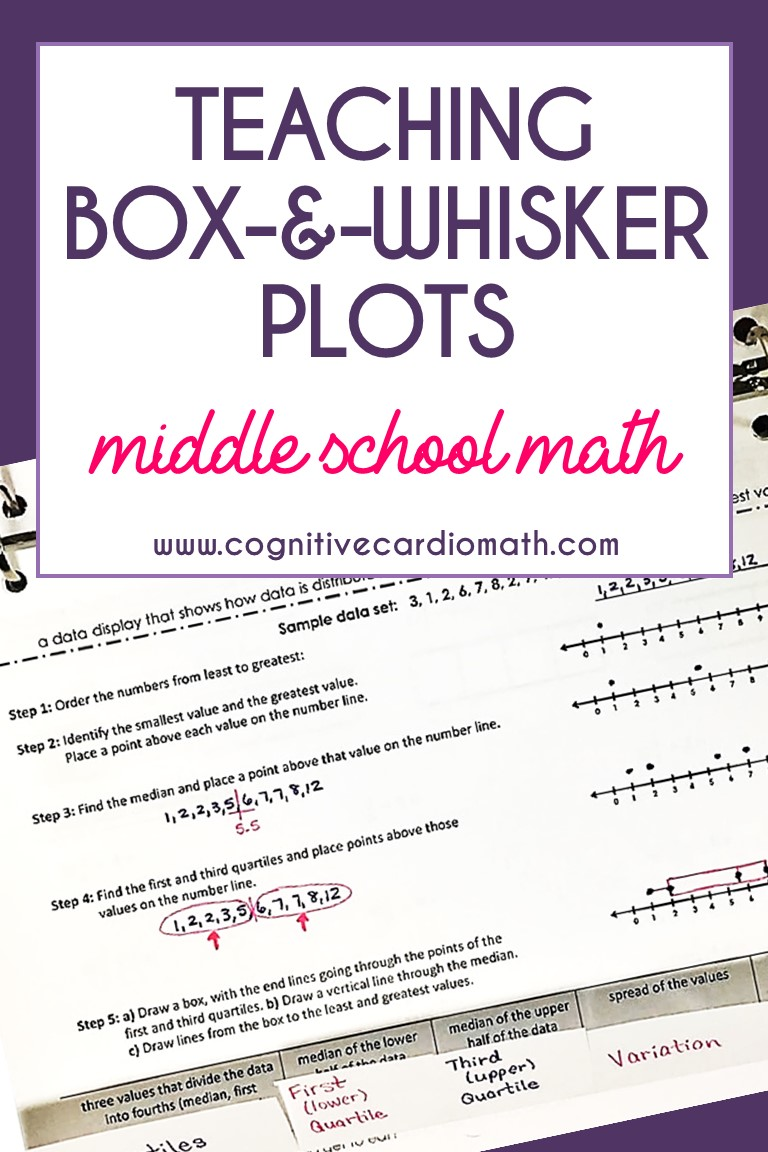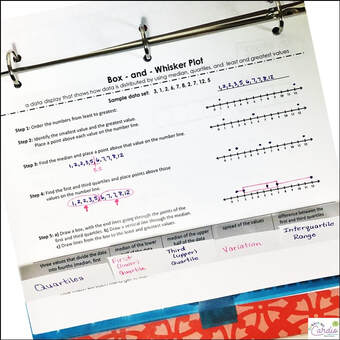# Teaching Box-and-Whisker Plots

## Teaching Box-and-Whisker Plots in 6th Grade Math

Box-and-whisker plots are a brand new concept for my 6th-grade math students, and when 6th graders are first introduced to them, they seem a little scary.

However, with some structured directions, students catch on very quickly.

As with all math concepts, there are different approaches to teaching this one.What has worked in my classes is this:

I break the creation of the box-and-whisker plot into 5 steps, in order to plot the 5 points needed to create the box and whiskers:

1) Order the data set from least to greatest.

2) Identify the smallest and largest values; place those points on the number line (above the number line).

3) Identify the median and place that point on the number line.

• Students need to understand and remember that if there’s an even number of numbers in the data set, the median will be the mean of the two middle numbers. Even though they’ve found median in the past, many students tend to need this reminder.

4) Find the first and third quartiles and place those points on the number line.

This step can be tricky for students…I’ve found it to be the step that most often throws off their box.

• Here’s what I’ve noticed: when there’s an odd number of numbers in the data set, it’s a little easier for students – the median is not included in the upper and lower halves of the data, so they have no trouble with circling the halves on either side of the median and then finding the median of each half, as in figure 1.
• When the data set has an even number of numbers, students need to remember that the median is between numbers and that exactly half of the numbers are in the upper half of the data and half are in the lower, as shown in figure 2.

The common mistake students make is to assume that since the two middle numbers (in this case 6 and 7) were used to find the median, they aren’t part of the upper and lower halves.

This throws off their 1st and 3rd quartiles. I’ve found it’s important to spend extra time on the different scenarios possible in this step. I’ve also found that drawing that middle line to represent the median is a very helpful visual.

5) Draw the box and whiskers:
a) Draw a box, with the (vertical) end lines going through the points of the first and third quartiles.
b) Draw a vertical line through the median.
c) Draw lines from the box to the least and greatest values.

## Box-and-Whisker Plot Fold It Up

I created this notes/fold-it-up a couple years ago, to help guide my students as they began creating box-and-whisker plots on their own.

There are two versions: a blank one for students and a completed one for me:-)(If students miss the instruction due to absence, I give them a copy of my completed version when they return.)

Using the blank version, I walk the students through their creation of the box-and-whisker plot. And when we’re done, they have the notes for their independent work.

The only folding part of this fold-it-up is the section at the very bottom that has the definitions of the vocabulary.

Students will need to cut the vertical lines along the bottom, fold them up, and then write the vocabulary words on the outside of the flaps

• quartiles
• first quartile
• third quartile
• variation
• interquartile range

## Box-and-Whisker Plots Notes and Practice

I recently created a box-and-whisker math wheel

It has the same steps outlined above, just in a different format, which is a little more colorful and fun! Both of these resources are great for interactive notebooks!Need more Fold It Ups? Check out these blog posts:

## Box and Whisker Plot Practice

If you need some additional, engaging practice for box-and-whisker plots, check out this color by number activity!

The problems in this activity require students to identify:

• First quartile
• Median
• Third quartile
• Interquartile range• Range
• Minimum
• Maximum
• Number that would be in each quartile, based on a number of students surveyed
• Data in terms of percent (25%, 50%, 75%
I hope you enjoy teaching box-and-whisker plots!

## EllieWelcome to Cognitive Cardio Math! I’m Ellie, a wife, mom, grandma, and dog ‘mom,’ and I’ve spent just about my whole life in school! With nearly 30 years in education, I’ve taught:

• All subject areas in 4th and 5th grades
• Math, ELA, and science in 6th grade (middle school)

I’ve been creating resources for teachers since 2012 and have worked in the elearning industry for about five years as well!